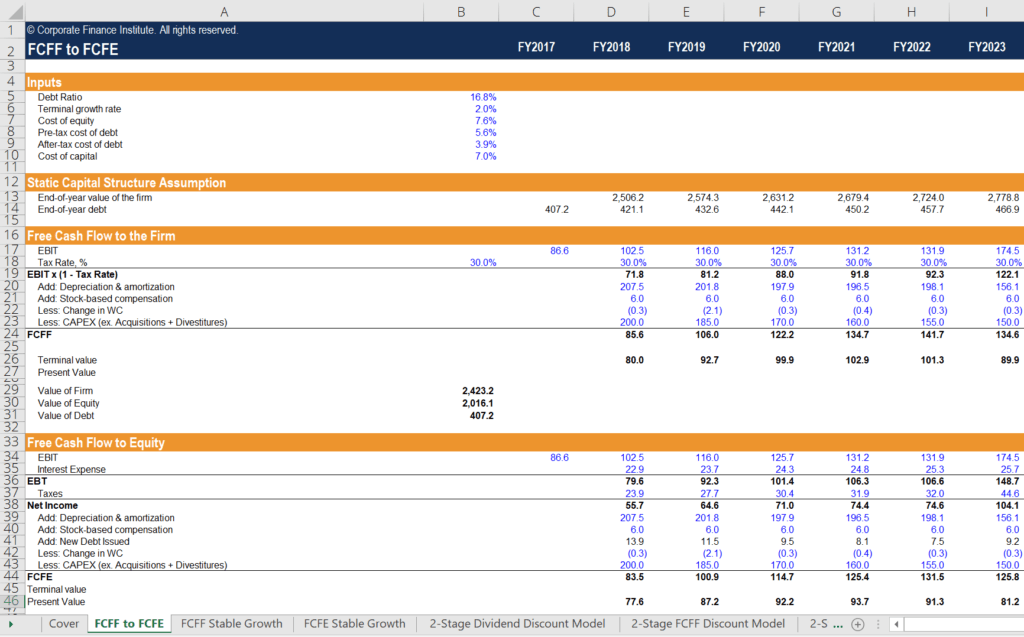# FCFF vs FCFE vs Dividends

Different ways to discount cash flows

## FCFF vs FCFE vs Dividends

All three types of cash flow – FCFF vs FCFE vs Dividends – can be used to determine the intrinsic value of equity, and ultimately, a firm’s intrinsic stock price. The primary difference in the valuation methods lies in how the cash flows are discounted. All three methods account for the inclusion of debt in a firm’s capital structure, albeit in different ways. Utilizing the provided worksheet, we can illustrate how the different types of cash flows (FCFF vs FCFE vs Dividends) reconcile, how they are valued, and when each type is most appropriate for valuation.Free cash flow to the firm (FCFF) is the cash flow available to all the firm’s suppliers of capital once the firm pays all operating and investing expenditures needed to sustain its existence. Operating expenditures include both variable and fixed costs necessary to generate revenues. Investing activities include expenditures by a company on its property, plant, and equipment. They also include cost of intangible assets, along with short-term working capital investments such as inventory. Also included are the deferred payments and receipts of revenue in its accounts payable and receivable. The remaining cash flows are those that are available to the firm’s suppliers of capital, namely its stockholders and bondholders.

Free cash flow to equity (FCFE) is the cash flow available to the firm’s stockholders only. These cash flows are inclusive of all of the expenses above, along with net cash outflows to bondholders. Using the dividend discount model is similar to the FCFE approach, as both forms of cash flows represent the cash flows available to stockholders. Among the FCFF vs FCFE vs Dividends models, the FCFE method is preferred when the dividend policy of the firm is not stable, or when an investor owns a controlling interest in the firm.

### Reconciling FCFF with FCFE

To reconcile FCFF with FCFE, we must make important assumptions about the firm’s financials and capital structure. First, we must assume that the capital structure of the firm will not change over time. This is an important assumption because if the firm’s capital structure changes, then the marginal cost of capital changes. Second, we must work with the same fundamental financial variables to both methods. Finally, we must apply the same tax rates and reinvestment requirements to both methods.#### Steps

1. Enter the base inputs of the calculation worksheet. These include the firm’s debt ratio (which is assumed to remain static), the pre-tax cost of debt, the tax rate, the cost of equity and the terminal growth rate.
• The free cash flow to the firm is determined in each year by converting the company’s operating profit (EBIT) to NOPAT by multiplying by (1 – tax rate), adding back non-cash expenses and subtracting net firm reinvestment (working capital and capital expenditures).
• The present value of the firm’s FCFF and terminal value are added together to find the intrinsic value of the firm as of today. Assuming the company has zero cash, subtracting the value of debt from the firm’s valuation will yield the value of equity.
2. The static capital structure assumption section calculates the value of the firm in each respective forward year, using each forward year as the present year to calculate different present values. Then, the end-of-year debt assumption is computed by taking the product of each forward year’s firm value and the static debt to capital ratio. From this point, we can begin computing the firm’s equity value standalone.
• We begin with the firm’s operating profit (EBIT) and subtract the firm’s interest expense. The interest expense is calculated by taking the product of the firm’s cost of debt and its year-end debt in each forward year. The difference yields the firm’s earnings before tax (EBT).
• The tax expense is calculated by taking the product of the tax rate used in the FCFF section and the earnings before tax in each forward year. The difference yields the firm’s net income.
• The free cash flow to equity is computed by taking the firm’s net income in each forward year, adding back non-cash charges, and subtracting net firm reinvestment – just as in FCFF with one key difference. We must also add back the net increase in debt, as this is new capital that is available for the firm.
• The present value of the firm’s FCFE and the terminal value of its equity are added together to find the current intrinsic value of the firm.

### Insights on FCFF vs FCFE vs Dividends

The first thing we notice is that we arrive at the same equity valuation with both methods. The first difference in the two methods is the discount rate applied. The FCFF method utilizes the weighted average cost of capital (WACC), whereas the FCFE method utilizes the cost of equity only. The second difference is the treatment of debt. The FCFF method subtracts debt at the very end to arrive at the intrinsic value of equity. The FCFE method integrates interest payments and net additions to debt to arrive at FCFE.

### Other Resources

We hope you’ve enjoyed CFI’s analysis of FCFF vs FCFE vs Dividends. CFI offers the Financial Modeling and Valuation Analyst (FMVA) certification program, designed to transform anyone into a world-class financial analyst. To keep learning and developing your knowledge of financial analysis, we highly recommend the additional resources below:

• Capital Structure
• Cost of Equity
• Valuation Methods
• Weighted Average Cost of Capital (WACC)

### Financial Analyst Training

Get world-class financial training with CFI’s online certified financial analyst training program!

Gain the confidence you need to move up the ladder in a high powered corporate finance career path.

Learn financial modeling and valuation in Excel the easy way, with step-by-step training.